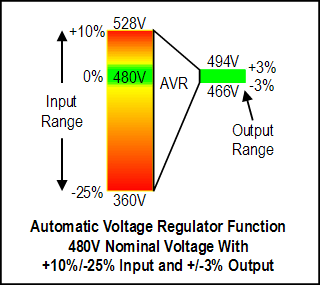#### AVR RegulationAn AVR takes in a range of voltage levels and automatically outputs a voltage with a much narrower range of voltage levels. For example, a typical automatic voltage regulator for power quality application may have an input voltage range of +10% to -25% of the nominal input voltage and convert this to a regulated voltage range of +3% to -3% of the nominal output voltage as shown the graphic for a 480V input and output. A voltage regulator may have a symmetrical input voltage range (e.g. +10% to -10% of nominal voltage) or an asymmetrical input voltage range as shown in the example. The choice of symmetrical versus asymmetrical input voltage range is dictated by purpose and design of the voltage regulator. The output voltage regulation range is almost universally symmetrical (e.g. +3% to -3% of nominal output voltage).

A voltage regulator may also perform a voltage step up or step down function whereby the nominal incoming voltage is transformed to a different output voltage level (e.g. a step up from a 208V input to a 400V output). For a voltage regulator with step up or step down capability, the input and output voltage ranges are usually applied to the input and output voltages (e.g. the example would become 208V +10/-25% on the input voltage range and 400V +3%/-3% for the regulated output range).